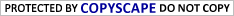## Program to find Factor of a number in C#

Write a program to find the Factor of a number entered by the user.

The basic approach to solve this problem is to find the number(s) (Factors) which can divide the number entered by the user with no remainder left i.e. 0. In simple words, a factor divides a number completely without leaving any remainder.

Program
:
 Console.WriteLine("Enter a Number"); //Get the Number from user for which factors needs to be calculate int num = int.Parse(Console.ReadLine()); Console.WriteLine("Factors are:"); //Start loop from 1 till the number entered by the user for (int i = 1; i <= num; i++) { //Check if Number is evenly into the number with no remainder (Remainder must be 0) //Then consider the number i.e. i as Factor     if (num % i == 0)     {     //Print the No. of Console     Console.WriteLine(i);     } } //Console.ReadLine() to hold the screen after the exection of the program Console.ReadLine();

Output:

Thanks